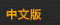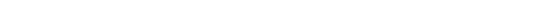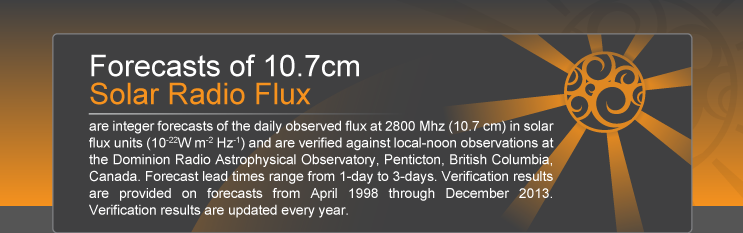﻿ Forecast VerificationYou are here: Home>Forecast Verification
Forecast VerificationVerification Statistics Summary Tables

Mean (f) Mean (o) Total Records Total number of forecast/observation records used to generate statistic Mean forecast Mean observation Median forecast Median observation Standard deviation of forecasts Standard deviation of observations Mean error Mean absolute error Mean relative error Root mean square error Mean absolute percent error Correlation coefficient between forecasts and observations Mean absolute error skill score Mean square error skill score

Forecast Type: F10.7 Solar Flux

Query:

Plot
1Forecast VS Observation Plot
2Forecast ME,MAE,MRE,MAPE Plot
3F10.7 Forecast Linear Association Plot
4Forecast Skill VS Lead Time
This plot shows SEPC forecast skill as a function of lead-time for forecasts of 10.7 cm solar flux. The skill of forecasts produced by SEPC is compared to that of forecasts produced by 27-day recurrence. Negative values indicate no skill above constant forecasts of sample recurrence.